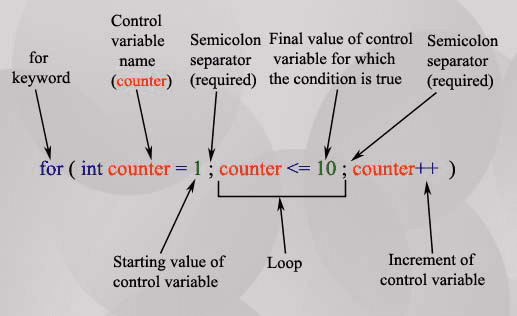## Description

A "For" Loop is used to repeat a specific block of code (statements) a known number of times. The for-loop statement is a very specialized while loop, which increase the readability of a program. Here is the syntax of the of for loop.

for ( initialize counter ; test counter ; increment counter)
{
execute the statement(s);
}
• initialize counter : Initialize the loop counter value.
• test counter : Verify the loop counter whether the condition is true.
• increment counter : Increasing the loop counter value.
• execute the statement : Execute C statements.

Note : The for-loop must have two semi-colons between the opening and closing parenthesis.

The following picture has clearly described the for loop syntax.## Why For Loops?

1. " For" loops execute blocks of code over and over again.

2. It is clear to an developer exactly how many times the loop will execute before the loop starts.

3. The Syntax of the for loop is almost same to other programming languages.

## For loop repetition statement

Here are some example of for loop repetition statements.

The following code prints the numbers from 1 to 100 in increments of 1.

for ( int x = 1; x <= 100 ; x++ )
{
printf("%d\n",x);
}

The following code prints the numbers from 100 to 1 in increments of -1.

for(int x = 100 ; x >= 1; x--)
{
printf("%d\n",x);
}

The following code prints the numbers from 8 to 88 in steps of 8

for(int x = 8; x <= 88 ; x += 8)
{
printf("%d\n",x);
}

The following code prints : 2, 7, 12, 17, 22, 27

for(int x = 2; x <= 30 ; x += 5)
{
printf("%d\n",x);
}

The following code prints: 66, 60, 54, 48, 42, 36, 30, 24, 18, 12, 6, 0

for(int x = 66 ; x >= 0; x -= 6 )
{
printf("%d\n",x);
}

## Example - 1

The following program calculate the sum of 1+2+3+...+50. The sum is stated in sum = sum + x, where i takes values from 1 to 50.

1. #include<stdio.h>
2. main()
3. {
4. int sum; int x; sum=0;
5.  for(x=1;x<=50;++x)
6.  // x take values in {1,2,3,...,50}
7.  {
8.  sum = sum + x;
9.  }
10.  printf(" 1+2+...+50=%d\n",sum);
11. }

1+2+...+50=1275

## Example - 2

The following program will ask the user to input 10 integers and find the sum.

1. #include<stdio.h>
2. main()
3. {
4. int z;
5. int x, sum=0, inpn;
6. // initialization
7. for(x=1; x<=10; ++x)
8. // Ask user 10 times(i.e. x takes 10 integers)
9. {
10. printf("Enter #%d: ",x);
11. scanf("%d",&inpn);
12. sum = sum + inpn;
13. // Accumulate the next value
14. }
15. printf("Total Sum of 10 numbers = %d\n",sum);
16. }

## Output :

Enter #1: 1
Enter #2: 1
Enter #3: 1
Enter #4: 1
Enter #5: 1
Enter #6: 1
Enter #7: 1
Enter #8: 1
Enter #9: 1
Enter #10: 2
Total Sum of 10 numbers = 11

## Example - 3

The following program will ask the user to input 5 numbers and print out the maximum and minimum numbers from the set.

1. #include<stdio.h>
2. main()
3. {
4. int Max, Min, Inpn, x;
5. printf("Input #1: ");
6. scanf("%d",&Inpn);
7. Max = Inpn;
8. Min = Inpn;
9. // Call the First number as current maximum and minimum
10. for(x=2;x<=5;++x)
11. {
12. printf("Input #%d: ",x);
13. scanf("%d",&Inpn);
14. if(Inpn>Max)
15. // if the next number is bigger than current maximum, store it
16. {
17. Max = Inpn;
18. }
19. if(Inpn<Min)
20. // if the next number is lower than current minimum, store it
21. {
22. Min = Inpn;
23. }
24. }
25. printf(" The Maximum # is %d\n",Max);
26. printf(" The Minimum # is %d\n",Min);
27. }

## Output :

Input #1: 120
Input #2: 34
Input #3: 0
Input #4: 1234
Input #5: -500
The Maximum # is 1234
The Minimum # is -500

## Example - 4

A prime number is a number that is only divisible by 1 and itself. We can check whether a number x is prime by checking if it is divisible by any of the numbers between 2 to x-1. For example if an user input a number say 5, then we will check if 5 is divisible by 2, 3 or 4. If 5 is divisible by 2, 3 or 4 then we can say that 5 is not prime. In the following program we use a for loop to iterate with y over the numbers 2,3,....x-1 and check if y is divided by the number x. Within if statement we use an indicator (z=1) to mark that x is not prime and during the for loop execution if the reminder of x and y is found 0 the break statement will cause the loop to exit.

1. #include<stdio.h>
2. main()
3. {
4. int x, z;
5. z=0;
6. printf("Input a number : ",x);
7. scanf("%d",&x);
8. for(int y = 2; y<x; y++)
9.    {
10.      if (x%y == 0)
11.       {
12.     // y divides x - not  a prime number
13.        printf("%d is not a prime number",x);
14.        z=1;
15.        break;
16.       }
17.     }
18. if (z==0)
19.  {
20.   printf("%d is a prime number",x);
21.  }
22. }

## Output :

Input a number : 7
7 is a prime number

Input a number : 8
8 is not a prime number

source:http://www.w3resource.com/c-programming/c-for-loop.php

﻿